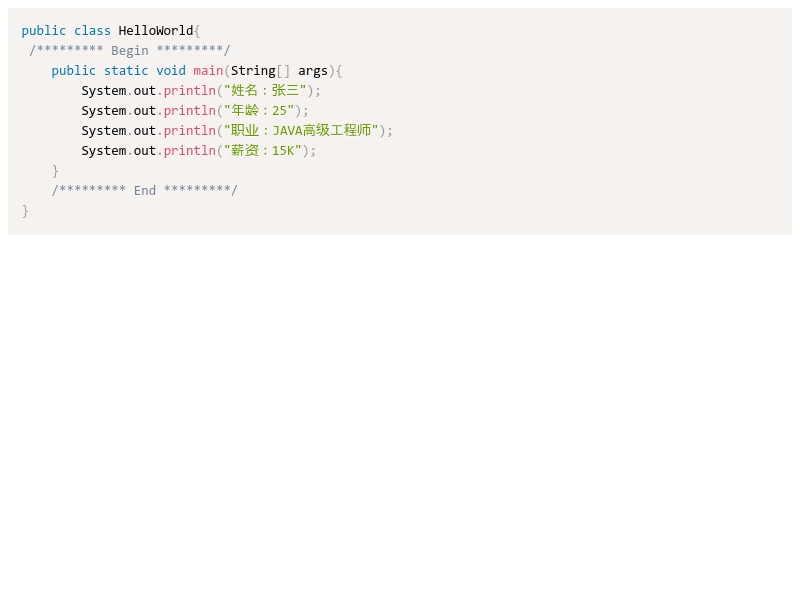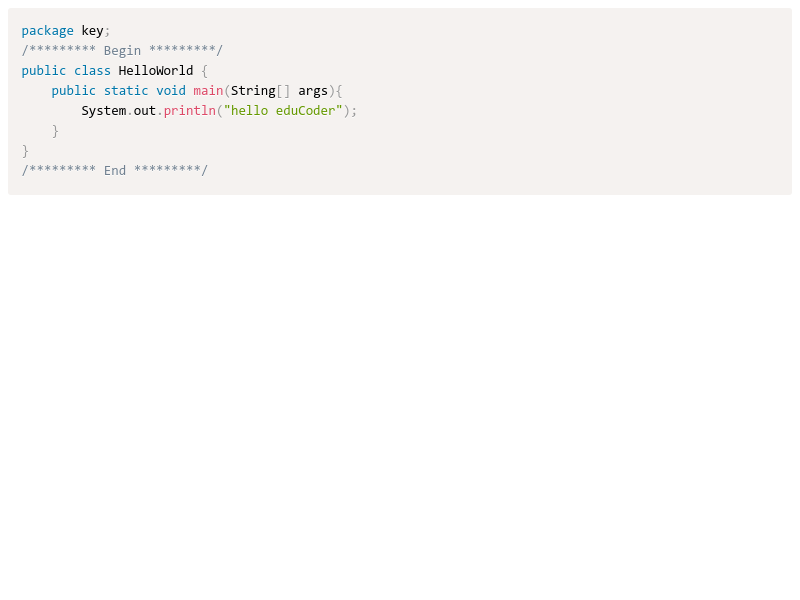• java入门测试题
2020-06-26 16:21:18

# 第2关：JAVA入门测试题

1、Java源代码文件的扩展名为（ ）
A 、.txt
B 、.class
C 、.java
D 、.doc

2、在控制台显示消息的语句正确的是（  ）
A 、System.out.println(我是一个Java程序员了！);
B 、System.Out.Println("我是一个Java程序员了！");
C 、System.out.println("我是一个Java程序员了！");
D 、System.Out.Println("我是一个Java程序员了！");

3、下面选项中不是开发Java程序的步骤（  ）
A 、编译
B 、编写源代码
C 、运行
D 、发布

4、在控制台编译并运行一个Java程序，使用的命令正确的是（    ）选两项
A 、java Test.java
B 、javac Test.java
C 、java Test
D 、java test


C
C
D
BC

# 第4关：JAVA关键字测试题

1、下面（  ）不是Java关键字
A 、Public
B 、string
C 、int
D 、num


## 答案

ABD


# 第6关：JAVA标识符测试题

1、以下（）是合法的标识符
A 、double
B 、3x
C 、sum
D 、de2\$f

2、有如下Java代码

        public class Demo{
public static void main(String[] args){
String title="hello";
System.out.println(title);
int %hour=18;
String name="张三",Name="李四";
}
}

有错误的行号是
A 、2
B 、3
C 、4
D 、5
E 、6


## 答案

CD
D

更多相关内容
• java基础入门第二版，针对每一个所讲解的知识点都进行了深入地分析，并使用生动形象的情境化举例，将原本复杂的、难于理解的知识点和问题进行简化，真正遵循了由浅入深、由易到难的学习过程。另外，针对每个知识点，...
• 简介 其他各类实训答案的目录见这里 答案查询的入口网页版 答案获取的方法简介见这里 ...Java入门 - Java初体验 >>>查看 第1关:Java第一课 第3关:JAVA关键字 第5关:JAVA标识符 第7关:JAVA注释 ...

## Java入门 - Java初体验 >>>查看

### 第1关:Java第一课### 第3关:JAVA关键字### 第5关:JAVA标识符

展开全文开发语言 后端
• 数据结构是计算机存储、组织数据的方式。数据结构是指相互之间存在一种或多种特定关系的数据元素的集合。通常情况下，精心选择的数据结构可以带来更高的运行或者存储效率。数据结构往往同高效的检索算法和索引技术...
• 直接上，如下： 1. class Happy {  public static void main(String args[]) {  int i = 1 ;   int j = i++ ;  if((i==(++j))&&((i++)==j)) {  i += j ;  }
直接上题，如下：
1.
class Happy {
public static void main(String args[])     {
int i = 1 ;
int j = i++ ;
if((i==(++j))&&((i++)==j))     {
i += j ;
}
System.out.println("i = "+i);
}
}
运行完上面代码之后输出i的值是多少？
A. 4
B. 5
C. 3
D. 6
2. 下面的数据声明及赋值那一个是没有错误的？
A. float f = 1.3;
B. char c = "a"
C. byte b = 257
D. int i = 10
3. 编译Java源程序文件产生的字节码文件的扩展名为？
A. java
B. class
C. html
D. exe
4. 现在假设有如下程序：
public class Demo {
public static void main(String args[]) {
boolean flag = 10%2 == 1 && 10 / 3 == 0 && 1 / 0 == 0 ;
System.out.println(flag ? "aliyunedu" : "yootk") ;
}
}
以上程序的最终执行结果是什么？
A. aliyunedu
B. yootk
C. true
D. 程序出错
5. 现在假设有如下程序：
public class Demo {
public static void main(String args[]) {
int x = 10 ;
double y = 20.2 ;
long z = 10L;
String str = "" + x + y * z ;
System.out.println(str) ;
}
}
以上程序的最终执行结果是什么？
A. 10202.0
B. 0212.0
C. 302.0
D. 1020.210
6. 现在假设有如下程序：
public class Demo {
public static void main(String args[]) {
String str = "" ;
for (int x = 0 ; x < 5 ; x ++) {
str += x ;
}
System.out.println(str) ;
}
}
以上程序最终的执行结果是什么？
A. 01234
B. 10
C. 14
D. 25
7. 现在假设有如下程序：
public class Demo {
public static void main(String args[]) {
System.out.println(inc(10) + inc(8) + inc(-10)) ;
}
public static int inc(int temp) {
if (temp > 0) {
return temp * 2 ;
}
return -1 ;
}
}
以上程序的最终执行结果是什么？
A. 35
B. 8
C. 28
D. 12
8. 现在假设有如下程序：
public class Demo {
public static void main(String args[]) {
char c = 'A' ;
int num = 10 ;
switch(c) {
case 'B' :
num ++ ;
case 'A' :
num ++ ;
case 'Y' :
num ++ ;
break ;
default :
num -- ;
}
System.out.println(num) ;
}
}
以上程序的最终执行结果是什么？
A. 11
B. 13
C. 12
D. 10
9. 现在假设有如下程序：
public class Demo {
public static void main(String args[]) {
int sum = 0 ;
for (int x = 1 ; x < 10 ; x ++) {
sum += x ;
if (x % 3 == 0) {
continue ;
}
}
System.out.println(sum) ;
}
}
以上程序的最终执行结果是什么？
A. 6
B. 0
C. 程序错误，死循环
D. 45
10. 现在假设有如下程序：
public class Demo {
public static void main(String args[]) {
int sum = 0 ;
for (int x = 0 ; x < 10 ; x ++) {
sum += x ;
if (x % 3 == 0) {
break ;
}
}
System.out.println(sum) ;
}
}
以上程序的最终执行结果是什么？
A. 6
B. 0
C. 程序错误，死循环
D. 45
答案：BDBBA AACDB
你做对了多少呢？若想学习请到 http://click.aliyun.com/m/23565/

本文转载自：阿里云云栖社区更多技术干货及好文，请关注
官方微博：@阿里云云栖社区   现在有免费赠手机的活动哦
官方微信：云栖社区（yunqiinsight）
官方知乎号：阿里云云栖社区
展开全文云栖社区 阿里云
• java基础测试题带答案导读：小编根据大家的需要整理了一份关于《java基础测试题带答案》的内容，具体内容：单选题：(每道题目2分)1、下列哪个声明是错误的？(B)A、inti=10;B、floatf=1.1;//floatf=1.1fC、do...单选...

java基础测试题带答案

导读：小编根据大家的需要整理了一份关于《java基础测试题带答案》的内容，具体内容：单选题：(每道题目2分)1、下列哪个声明是错误的？(B)A、inti=10;B、floatf=1.1;  //floatf=1.1fC、do...

单选题：(每道题目2分)

1、下列哪个声明是错误的？(B)

A、 int i=10;

B、 float f=1.1;     //float f=1.1f

C、 double d=34.4;

D、 byte b=127;

long类型的数据加后缀L或者l

float类型的数据加后缀F或者f

整数默认是int类型

浮点数默认是double类型

2、下面哪个不是java中的关键字？(C)

A、public

B、 true

C、 main

D、 class

3、下面程序哪个语句是正确的C

A、 byte a=0,b=3; byte c =a+b;//a+b的类型为int

B、 short s =23; s=s+12;

//s+12底层是转成int进行相加，所以结果为int。

C、 short s=23; s+=12;  //相当于s=shorts+12

D、 float f = 23+23.23;  //23+23.23的结果为double

4、下面程序执行的结果是？B

class  Test

{

public static  void  mainString[] args

{

System.out.println“”+‘a’+1;

}

}

A、98

B、 a1

C、 971

D、 197

//空串放前相当于字符串的拼接，空串放后，‘a’+1，先做运算，再与空串进行拼接，byte,short,char可以自动转为int,

5、下面程序执行的结果是？(B)

int i =100;

whiletrue

{

if i++ > 100   //i先计算在自加

{

break;

}

System.out.printlni;

}

A、 100

B、 101

C、 102

D、 报错

6、 下面程序的运行结果是   D

int a=3,b=1;

ifa==b

{

System.out.println"a="+a;

}

A、 a=1

B、 a=3

C、 编译错误

D、 正常运行但没有输出

7、下面程序的运行后,结果正确的是：(B)

inta=1,b=2;

intc=a+b>3?a++:++b; //a=1,b=3,c=3

A、 a=2,b=3

B、 a=1,b=3

C、 a=1,b=2

D、 c=2

8、下面程序的运行结果(B)

classDemo

{

public static int funint c

{

return c+=2;

}

public static void mainString[] args

{

int temp = fun2;

System.out.printlntemp;

}

}

A、 2

B、 4

C、 6

D、 8

9、下面数组定义错误的是(C)

A、 int [] arr ={23,45,65,78,89};  //静态初始化

B、 int [] arr=new int ;        //动态初始化

C、 int [] arr=new int{3,4,5,6};

D、 int [] arr={‘a’, 23 , 45 , 6}；

//‘a’可以自动转为int，

10、下面程序执行的结果是？(D )

int x=1,y=1;

ifx++==2& ++y==2

//x=2,y=2,&与&&的.结果相同但是&不具有短路效果

{

x=7;

}

System.out.println"x="+x+"  , y="+y;

A、 x=1 y=2

B、 x=7 y=1

C、 x=7 y=2

D、 x=2 y=2

11、下面不属于基本数据类型的是(D)

A、 int

B、 double

C、 long

D、 int[] //引用类型

12、下面程序执行的结果是？( C)

booleanb=true;

ifb=false         //将false赋值给b，则b=false

{

System.out.println"a";

}

elseifb

{

System.out.println"b";

}

elseif!b

{

System.out.println"c";

}

else

{

System.out.println"d";

}

A、a

B、b

C、c

D、d

13、下面程序执行的结果是？(D)

intx=2,y=3;

switchx

{

default:

y++;    //y=4,但是没有break,程序继续向下执行

case 3:

y++;

case 4:

y++;

}

Sysetem.out.println"y="+y;

A、 3

B、 4

C、 5

D、 6

14、下面程序执行的结果

forint  i=1;i<=10;i++

{

if i%3==0

{

continue;    //continue跳出本次循环

}

System.out.println“java基础班”;

}

在屏幕上打印几次“java基础班”? C

A、 5

B、 6

C、 7

D、 8

15.阅读下面代码段：

classDemo

{

public static void main String[] args

{

int[] arr = new int;

System.out.printlnarr;

}

}

执行结果正确的说法是 C

A、 编译时将产生错误

B、 编译时正确，运行时将产生错误

C、 输出零

D、 输出空

16 .和下面代码能完成相同的选项是(B)

int i=1;

intsum=0;

whilei<=100

{

ifi%2==0

{

sum=sum+i;

}

i++;

}

A、 for int x=1; x<=100;x++{ sum=sum+x;}

B、 for int x =0; x<=100;x+=2{ sum=sum+x;}  //偶数求和

C、 for int x =1; x<=100;x+=2{ sum=sum+x;}  //奇数求和

D.上述全对

17、以下代码输出是(  D )

int i=0;

int sum=0;

whilei<=10

{

i++;

if i%2!=0

continue;

sum+=i;

}

System.out.printlnsum;    //sum=2+4+6+8+10

A、 55

B、 45

C、 35

D、 30

18、给出下列代码片段:

if x> 0 { System.out.println“Hello”; }

else if x >-3 { System.out.pirntln “I am Tom”; }

else {System.out.println “How are you?”; }

请问将打印字符串 “How are you ?”的x的范围是( C)

A、  x>0

B、  x > -3

C、  x <= -3

D、  x <=0 x="">-3

19、下列代码执行的结果为 A

classDemo

{

public static void mainString[] args

{

int num =max43,34;     //num=43

System.out.printlnnum;

}

public static int maxint a,int b

{

returna>b?a:b;

}

}

A、 43

B、 23

C、 77

D、 9

20、下面程序执行的结果是 A

classDemo

{

public static void mainString [] args

{

int a=10;

ifa++>10

{

a=20;

}

System.out.printlna;

}

}

A、 11

B、 12

C、 20

D、 21

展开全文• , 《Java基础入门》附有配套视频、源代码、测试题、教学PPT、教学实施案例、教学设计大纲等资源，并提供在线答疑平台。, 本书既可作为高等院校本、专科计算机相关专业的程序设计课程教材，也可作为Java技术基础的...
• Java入门经典》按照学习Java的最佳顺序来分配章节，共...《Java入门经典》光盘提供了明日科技数十年的资源积累的程序开发资源库，包括海量实例资源库、模块资源库、项目案例资源库、测试题库系统、面试资源库等内容。
• 本资源是Java入门到项目实战【配套资源】自测题目，有丰富的Java测试题，选择、填空、简答均包含在内，适合Java开发者研究以及Java学习者的自我检测，对Java开发岗的面试也非常有帮助。
• 都是一些非常非常基础Java入门学习的，是我最近参加各大IT公司笔试后靠记忆记下来的，经过整理献给与我一样参加各大IT校园招聘的同学们，但是IT公司就喜欢考这些基础的东西，所以为了能进大公司就~~~当复习期末考...
•学习
• 文章目录Java 基础Head First JavaJava 核心技术卷一Java 编程思想设计模式Head First 设计模式图解设计模式设计模式重学 Java 设计模式Java 进阶Java 并发编程实战Java 并发编程艺术Java 并发编程之美图解Java多...
• 第1关：Java第一课 任务描述 本关任务：编写你的第一个Java程序，效果图如下： 编写第一个Java程序 1.新建一个HelloWorld.java文件（新建HelloWorld.txt将后缀.txt改成.java就ok了 注意：Java对大小写敏感所以大小...
• 这是入行10多年自己经历积累和网友分享的java面试，包《进入IT企业必读的324个JAVA面试》、《java名企面试》、《100IT名企java面试必考面试》等文档和面试讲解视频，总共539M，解压后将近1GB的面试材料。
• ## JAVA入门基础测试题

千次阅读 2017-06-22 11:43:24基础
• JAVA小白编程练习 可能有很多刚入门的小白不知道自己如何能快速提升编程技巧与熟练度 其实大佬进阶之路只有一个~ 那就是疯狂码代码!!!实践出真知!!! 所以为了大家能够想练习的时候有素材,泡泡给大家整理了一些练习...笔试 基础...